Author Message
Martin_K

Joined: 09 Apr 2020
Posts: 227Posted: Sun Apr 04, 2021 1:39 pm    Post subject: labelling of graphic objects in the %pl graphics regionI would like to label selected objects in the %pl graphics region with
their corresponding most important property.

The plot looks like this: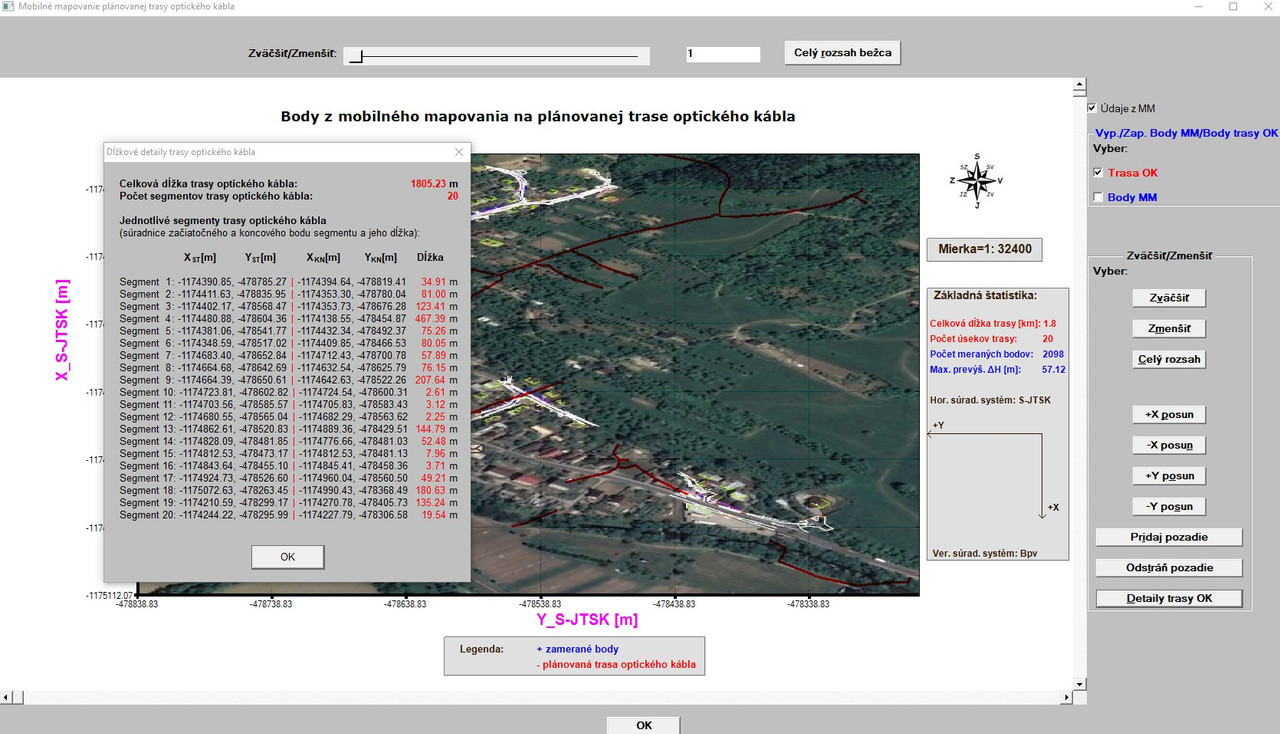I coded and added a function which identifies the starting and ending point of each line segment, computes length for each line segment, computes total length and displays this complex info in an info window as demonstrated above.

From this plot, however, cannot be seen where is located segment1,2, ...

Now, I would like to draw this "new" points (start and end points for each line segment) again to the plot with a point symbol and a different color
(these - in this case 40 - points are - in fact - already in the plot, but are invisible, since they are part of red lines).

So, I could append this "new" array to an existing one with %pl [stacked] option and then draw them this time as points with different symbol and color within
an existing code for %pl:

 Code: iw=winio@('%`^pl[full_mouse_input]&',gw,gh,n_pl,Y_OK_U,X_OK_U,handle_pl_ok,pl_cb)

where to the arrays Y_OK_U, X_OK_U would be added the new one and also N_PL would be added by +1.

Then, I would also add a check/uncheck box (to see it or not).

However, I would like to try a new approach (if it is even possible) by using the
function DRAW_POINT@, where I would use the GET_PLOT_POINT@ for the new array to get their pixel coordinates and draw them (the pixels)
in different color.

At the end, I would like to draw above/below each segment its ID (1,2,...) along with (maybe) its corresponding length.

But, I do not know, whether such approach is even possible and whether it is possible to tell the function DRAW_POINT@ where to draw the points.

Q: HANDLE_PL_OK graphics region should be selected first using SELECT_GRAPHICS_REGION@? or should it be created a new graphics
region, then drawing would occur in it and then to copy this region back
to the required one (handle_pl_OK) using copy mode 8913094?
And - if such approach would function, should it be placed within %pl callback or within %pl plot function itself?

Is there also a function like DRAW_POINT@, where would it be possible
to tell it which graphical symbol should be used for point to be drawn or this functionality
is exclusively available only among %PL options?

I am looking for the quickest and as simple as possible way how to achieve it (probably, the STACKED option will win, nevertheless - if there
is possible another approach, I would like to exetend my knowledge).

MartinKenneth_SmithJoined: 18 May 2012
Posts: 505
Location: Hamilton, Lanarkshire, Scotland.Posted: Sun Apr 04, 2021 5:36 pm    Post subject:Without use of %pl callback.

 Code: winapp module demo2_mod use clrwin implicit none integer, parameter :: dp=kind(1.d0) integer, parameter :: gw = 400, gh = 400, mtop = 50, mbottom = 50, mleft = 100, mright = 40 integer, parameter :: n = 6 real(kind=dp) :: x(1:n) = (/ (i-1 ,i=1,n) /), y(1:n) = (/ ((i-1)**2, i=1,n) /) contains integer function plot_data() integer iw character(len=126) pl_str   iw = winio@('%bg&',rgb@(240,240,250))   iw = winio@('%fn[Consolas]&')   iw = winio@('%2.1ob[invisible]&')   call winop@('%pl[native,x_array,independent,frame,etched,gridlines,width=4,colour=blue,smoothing=4]')   write(pl_str,'("%pl[margin=(",I3,",",I3,",",I3,",",I3,")]")') mleft, mtop, mright, mbottom   call winop@(pl_str)   iw = winio@('%^pl&',gw,gh,n,x,y,pl_cb)   iw = winio@('%cb&')   iw = winio@('%^tt[Toggle points]&',toggle_points_cb)   iw = winio@('%cb&')   iw = winio@('%ff%nl%cn%bn[OK]&')   iw = winio@(' ')   plot_data = 1 end function plot_data     integer function pl_cb()   pl_cb = 2 end function pl_cb     integer function toggle_points_cb() integer i, j real(kind=dp) xx,yy logical, save :: toggle = .false.   !OFF   if (.not. toggle) then     toggle = .true.   else     toggle = .false.   end if   if (toggle) then     do i = 1, n, 1       j = GET_PLOT_POINT@(x(i),y(i),xx,yy)       call draw_filled_ellipse@(nint(xx), nint(yy), 4, 4, rgb@(0,0,255))     end do   else     call simpleplot_redraw@()   end if   toggle_points_cb = 2 end function toggle_points_cb end module demo2_mod program main use demo2_mod i = plot_data() end program mainKenneth_SmithJoined: 18 May 2012
Posts: 505
Location: Hamilton, Lanarkshire, Scotland.Posted: Sun Apr 04, 2021 5:38 pm    Post subject:%rb + %pl callback
 Code: winapp module demo_mod use clrwin implicit none integer, parameter :: dp=kind(1.d0) integer, parameter :: gw = 400, gh = 400, mtop = 50, mbottom = 50, mleft = 100, mright = 40 integer, parameter :: n = 6 real(kind=dp) :: x(1:n) = (/ (i-1 ,i=1,n) /), y(1:n) = (/ ((i-1)**2, i=1,n) /) integer :: control_show_points = 0 contains integer function plot_data() integer iw character(len=126) pl_str   iw = winio@('%bg&',rgb@(240,240,250))   iw = winio@('%fn[Consolas]&')   iw = winio@('%2.1ob[invisible]&')   call winop@('%pl[native,x_array,independent,frame,etched,gridlines,width=4,colour=blue,smoothing=4]')   write(pl_str,'("%pl[margin=(",I3,",",I3,",",I3,",",I3,")]")') mleft, mtop, mright, mbottom   call winop@(pl_str)   iw = winio@('%^pl&',gw,gh,n,x,y,pl_cb)   iw = winio@('%cb&')   iw = winio@('%`^rb[Show points]&',control_show_points, update_cb)   iw = winio@('%cb&')   iw = winio@('%ff%nl%cn%bn[OK]&')   iw = winio@(' ')   plot_data = 1 end function plot_data     integer function pl_cb() integer i, j real(kind=dp) xx, yy   if (clearwin_string@('CALLBACK_REASON').eq.'PLOT_ADJUST') then     if (control_show_points .eq. 1) then       do i = 1, n, 1         j = GET_PLOT_POINT@(x(i),y(i),xx,yy)         call draw_filled_ellipse@(nint(xx), nint(yy), 4, 4, rgb@(0,0,255))       end do     end if   end if   pl_cb = 2 end function pl_cb integer function update_cb()   call simpleplot_redraw@()   update_cb = 2 end function update_cb end module demo_mod program main use demo_mod i = plot_data() end program main

Both of these examples illustrate how to determine the pixel coordinates at a data point and draw a filled circle at that point. This could be expanded to include data at each point using DRAW_CHARACTERS@(). The second approach is probably most appropriate for the existing code you have previously shared with us here.

In the second example, if you were using a %dl callback (for example to process scrollbar movements), then you don't need to attach a callback to the %rb. As shown below.Kenneth_SmithJoined: 18 May 2012
Posts: 505
Location: Hamilton, Lanarkshire, Scotland.Posted: Sun Apr 04, 2021 6:04 pm    Post subject:Code: winapp module demo_mod use clrwin implicit none integer, parameter :: dp=kind(1.d0) integer, parameter :: gw = 400, gh = 400, mtop = 50, mbottom = 50, mleft = 100, mright = 40 integer, parameter :: n = 6 real(kind=dp) :: x(1:n) = (/ (i-1 ,i=1,n) /), y(1:n) = (/ ((i-1)**2, i=1,n) /) integer :: control_show_points = 0 contains integer function plot_data() integer iw character(len=126) pl_str   iw = winio@('%bg&',rgb@(240,240,250))   iw = winio@('%fn[Consolas]&')   iw = winio@('%2.1ob[invisible]&')   call winop@('%pl[native,x_array,independent,frame,etched,gridlines,width=4,colour=blue,smoothing=4]')   write(pl_str,'("%pl[margin=(",I3,",",I3,",",I3,",",I3,")]")') mleft, mtop, mright, mbottom   call winop@(pl_str)   iw = winio@('%^pl&',gw,gh,n,x,y,pl_cb)   iw = winio@('%cb&')   iw = winio@('%`rb[Show points]&',control_show_points)   iw = winio@('%cb&')   iw = winio@('%ff%nl%cn%bn[OK]&')   iw = winio@('%dl&', 1.d0, update_cb)   iw = winio@(' ')   plot_data = 1 end function plot_data     integer function pl_cb() integer i, j real(kind=dp) xx, yy   if (clearwin_string@('CALLBACK_REASON').eq.'PLOT_ADJUST') then     if (control_show_points .eq. 1) then       do i = 1, n, 1         j = GET_PLOT_POINT@(x(i),y(i),xx,yy)         call draw_filled_ellipse@(nint(xx), nint(yy), 4, 4, rgb@(0,0,255))       end do     end if   end if   pl_cb = 2 end function pl_cb integer function update_cb()   call simpleplot_redraw@()   update_cb = 2 end function update_cb end module demo_mod program main use demo_mod i = plot_data() end program mainMartin_K

Joined: 09 Apr 2020
Posts: 227Posted: Mon Apr 05, 2021 10:47 am    Post subject:Thanks Ken! I built-in the 2nd approach (%`rb+ %pl callback) and after small changes regarding the color and the size of the ellipse/circle the plot looks now like this: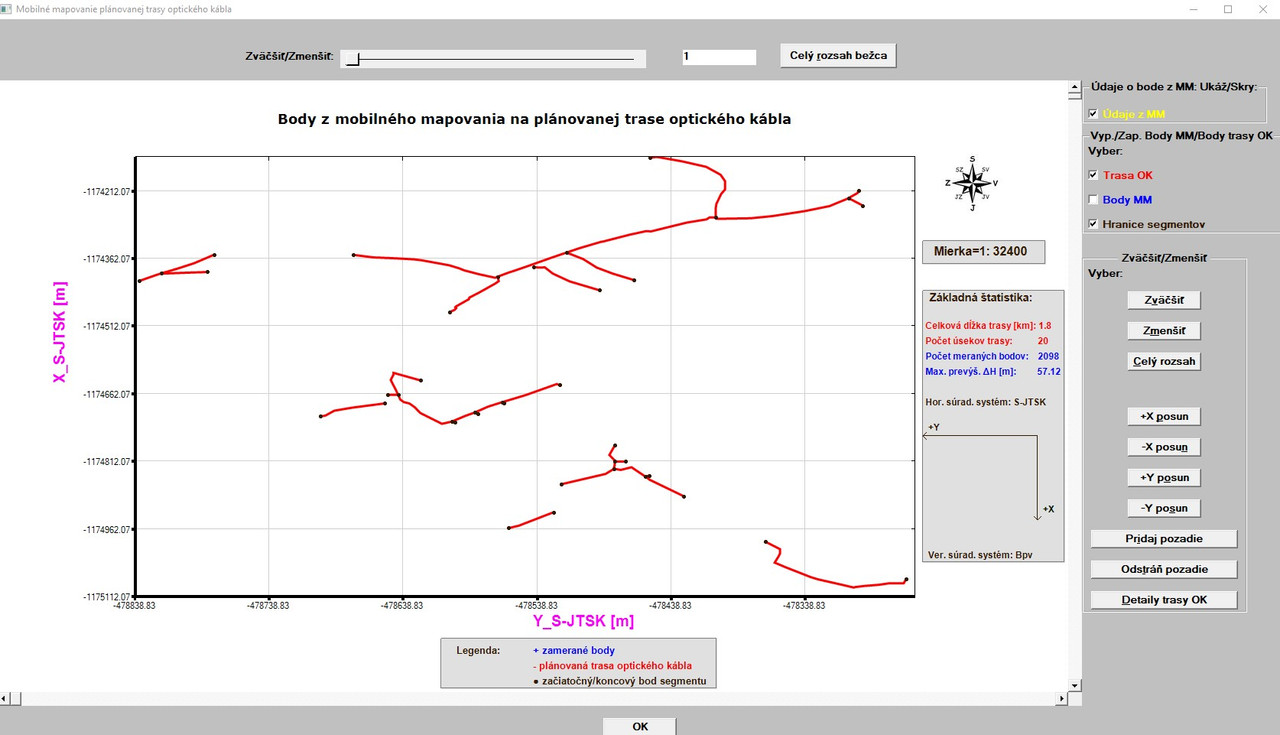Some remarks/questions/issues: Q1: Why did you use the NINT(XX) and NINT(YY)? I used their real values and if I compared the version with NINT and without NINT, in such scale I saw no difference. Nevertheless, I would like to know why you used NINT. Issue: When I zoom in the graph (regardless by which method, whether by the slider or by the button for zooming in, the start/end points of each line segment which lay near axes are also visible outside axes: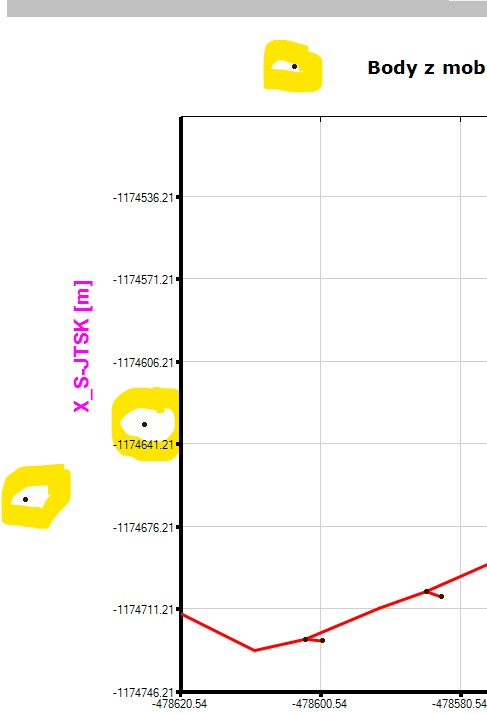Would also be the same issue when using the option STACKED? I have not such issue with STACKED when zooming in. Remark: I will introduce some logic to test where and which segment ID will be drawn (maybe along with its corresponding length) in the plot. I will probably take the X-coordinate of every starting point of each line segment for such testing and then will use DRAW_CHARACTERS@ with a small pixel offset for drawing of segment ID. However, it could be quite untransparent to draw such information (first of all, when there will also be their distances) on the segments which are very short as demonstrated here: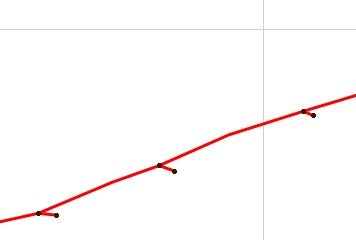Probably, it will be better to draw just segment ID (without its distance).Kenneth_SmithJoined: 18 May 2012
Posts: 505
Location: Hamilton, Lanarkshire, Scotland.Posted: Mon Apr 05, 2021 12:58 pm    Post subject:Martin,

My reason for using NINT can be seen in the following:-

SUBROUTINE DRAW_FILLED_ELLIPSE@(IXC,IYC,IA,IB,ICOL)
INTEGER IXC,IYC,IA,IB,ICOL

Of course you could instead use the new routine DRAW_FILLED_ELLIPSED@ which takes DOUBLE PRECISION values for co-ordinate points and sizes.

When using the drawing routines it is important to realise that these operations are performed after the %pl is redrawn with the current data, x and y ranges etc.

It should not be too difficult to test if a point is within the frame of the plot. You can do this using its physical coordinates and the current range (min and max) for the x and y axis (all real*8 values), or using it's pixel coordinates and the %pl margins (all integer values)

Here is a simple function for performing such a test with real*8 variables:
 Code: logical function inrange_d(x,a,b) ! Returns logic TRUE if x is in range defined by a and b inclusive otherwise FALSE real*8, intent(in) :: x,a,b   inrange_d = .false.   if ( ( x .ge. min(a,b) ) .and. ( x .le. max(a,b) ) ) inrange_d = .true. end function inrange_dMartin_K

Joined: 09 Apr 2020
Posts: 227Posted: Mon Apr 05, 2021 6:19 pm    Post subject:Thanks!

The reason for my question: I used DRAW_FILLED_ELLIPSED@, but then I tried DRAW_FILLED_ELLIPSE@ to see a difference (if any),
where I forgot to change the real arguments to integer and got no compiler complaint. All was working problem free.
The same, when I noticed it and manually changed the real arguments to INT for DRAW_FILLED_ELLIPSE@ using NINT..

Put it into another words: the use of real*8 arguments with
DRAW_FILLED_ELLIPSE@ got unnoticed by compiler.

This is just a notice, maybe the function DRAW_FILLED_ELLIPSE@ did it automatically (the change of argument type to the proper one - INTEGER).

Now, I have some issue with your logical function.

When I use the code:

 Code: IF((INRANGE_X).AND.(INRANGE_Y)) THEN        i = update_PL_limits () END IF

the compiler says:
error 1039 - FUNCTION name, INRANGE_X, used where not expected, perhaps missing '()'.

Is my code incorrect?

Forget my question above - I already found the problem, now it formally works!. But it does not produce the required result - the points - when zooming in - are still displayed outside the axes frame. Probably, some
other logic problem I have.

Last edited by Martin_K on Mon Apr 05, 2021 7:05 pm; edited 1 time in totalKenneth_SmithJoined: 18 May 2012
Posts: 505
Location: Hamilton, Lanarkshire, Scotland.Posted: Mon Apr 05, 2021 7:04 pm    Post subject:Simple example:-

 Code: winapp program main real*8 x,a,b logical test logical, external :: inrange_d do i = 1, 20, 1   x = 2.d0 * random@()-1.d0   a = 2.d0 * random@()-1.d0   b = 2.d0 * random@()-1.d0   test = inrange_d(x,a,b)   if (test)       print*, x,'in range     ',a, b   if (.not. test) print*, x,'not in range ',a, b end do end program main logical function inrange_d(x,a,b) ! Returns logic TRUE if x is in range defined by a and b inclusive otherwise FALSE real*8, intent(in) :: x,a,b   inrange_d = .false.   if ( ( x .ge. min(a,b) ) .and. ( x .le. max(a,b) ) ) inrange_d = .true. end function inrange_dKenneth_SmithJoined: 18 May 2012
Posts: 505
Location: Hamilton, Lanarkshire, Scotland.Posted: Mon Apr 05, 2021 7:34 pm    Post subject:If a scrollbar is moved, the new scroll position is processed by the %dl callback, changing x_min_now and x_max_now, at the end of the %dl callback there is a call to simpleplot_redraw@(), which then triggers the %pl callback with clrearwin_string@("CALLBACK_REASON") = "PLOT_ADJUST". In the call to logical function inrange_d(x,a,b) within the clrearwin_string@("CALLBACK_REASON") = "PLOT_ADJUST" section of the %pl callback, a and b need to correspond to x_min_now and x_max_now as used previously in the %dl call back function at CHANGE_PLOT_DBL@(id, 'x_min', 0, x_min_now) and CHANGE_PLOT_DBL@(id, 'x_max', 0, x_max_now). Same applies to the y-axis.Martin_K

Joined: 09 Apr 2020
Posts: 227Posted: Mon Apr 05, 2021 11:30 pm    Post subject:Ken - thanks! In the meantime I coded to display the Point ID for every start point (subscript ST) and end point (subscript KN in the info window) of each segment line and I added this information (which start/end points form a segment) to info window to identify them in the plot: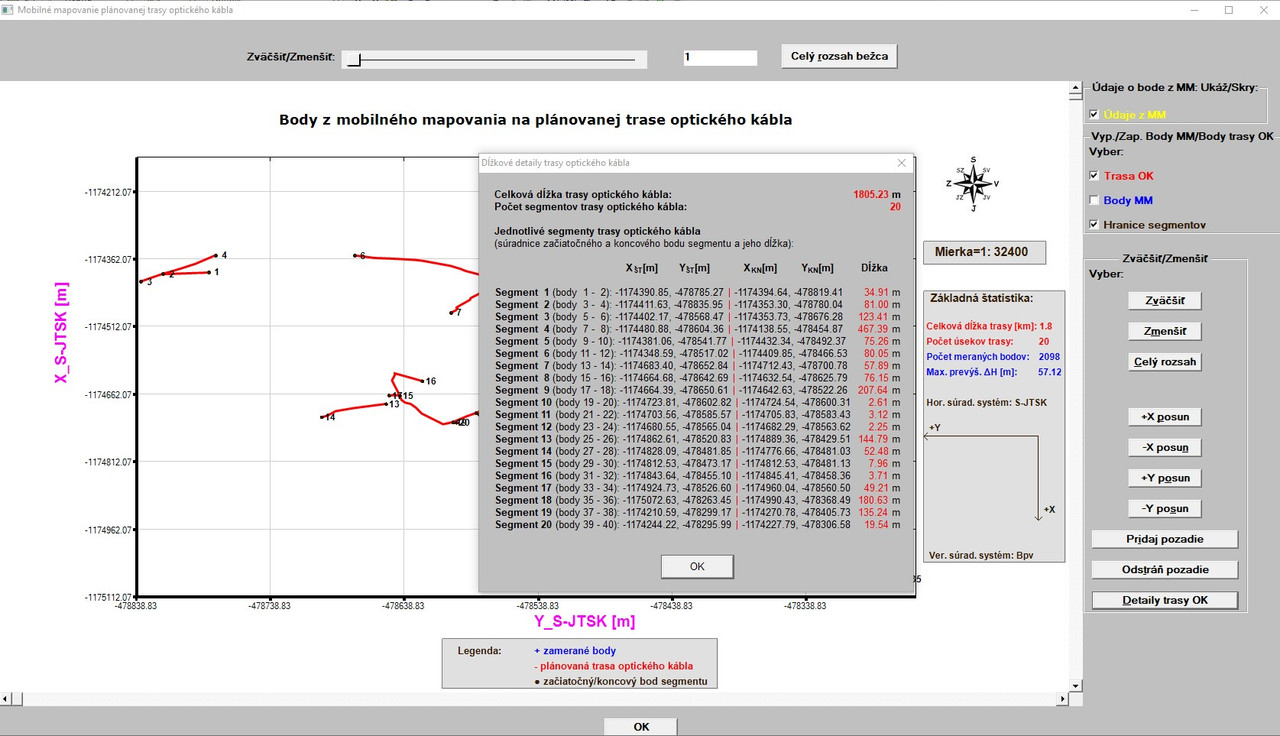This could be sufficient, since to add (to draw) the lengths of each segment in the plot would be - on one side very nice - but on the other side it would cause an untransparent plot, since there are many segments which are very short (2-3m in length) and there is no space for such information. But, I am still frustrated with the zooming in when the segment points are switched on in the plot. Although (at least I think so) I did it as you advised for the zooming in button, the maximum what I achieved is that I was able to zoom in it once, then no zoooming in was possible (only zoom out and to extents). So, at present stage all the start/end segment points still running out of the axes frame when zooming in. Probably, when I would use the STACKED option, there would be no such problem, but now, my code has about 2000 lines and every interference becomes quite dangerous for me that I mess up something what is working fine now. I will have a look at this once again tomorrow. Thanks again!Martin_K

Joined: 09 Apr 2020
Posts: 227Posted: Wed Apr 07, 2021 3:29 pm    Post subject:Ken,

I am unable to catch the problem with start/end points
which always (when zooming in with corresponding button
or when moving with slider) run outside the axis frame.

Could you have a look at the code of the %pl and %pl callback
please to see where am I wrong? - Thanks!

Here is the code:

https://www.dropbox.com/s/rscawofv5uv9npf/pl_function%2Bits_call-back%2Bfew_others.f95?dl=0

I have a question regarding %vx.

There is very high probability that when there are 50 or more line segments
available in the data set, the information window will exceed the height
of display and some part of the info window will not be visible.

I added the %vx option in the corresponding part of the code
(before the info window is created) as follows:

 Code: ...             iw=winio@("%vx&",vpage_step1,vmax_value1, vcur_val1)              w=0; h= 0               ans=winio@('%sz&',w,h) ...

However, no vertical scrollbar is attached to the window. Its callback I would define later, just now I wanted to see it in the window. Where am I wrong?

Thanks!Kenneth_SmithJoined: 18 May 2012
Posts: 505
Location: Hamilton, Lanarkshire, Scotland.Posted: Wed Apr 07, 2021 5:17 pm    Post subject:Martin,

I will look at your code when I have time, but it will not be today.

This might be a better solution to your second question, which is a slightly modified version of one of Paul's examples which I have found very useful for displaying long lists of tabulated data.

 Code: winapp module test_mod use mswin use clrwin integer wcontrol contains integer function gui() integer i i = show_list() gui = 1 end function gui integer function show_list() integer iw,winio@ integer(7) hwnd1, hwnd2 iw = winio@('%bg[grey]&') iw = winio@('%cn%ws&','Example of %cw with scroll for displaying lists') iw = winio@('%2nl%30.2cw&', 20) iw = winio@('%2nl%`bg[white]%`30.20cw[vscroll]&', 10, hwnd1) iw = winio@('%ff%nl%cn%^bn[OK]&', close_list_cb) iw = winio@('%lw&',wcontrol) iw = winio@(' ') write(20,*) write(20,'("Title  N   Data1   X")') do i = 1,200   write(10,'(A4,1X,I3,1X,F7.3,1X,I3)') "Line ", i, random@(), INT(100.d0*random@()) end do iw = SendMessage(hwnd1, WM_VSCROLL, SB_TOP, 0) end function show_list integer function close_list_cb()   wcontrol = 0   call window_update@(wcontrol)   close_list_cb = 2 end function close_list_cb end module test_mod program main use test_mod implicit none integer i i = gui() end program main

PS Meantime, I suggest you check that your code which draws the points is contained in an IF (TEST) THEN END IF BLOCK, where TEST confirms that either the physical coordinates are within the limits of the display (xmin etc), or that the pixel coordinates are within the pl frame (gw - leftmargin, etc).Martin_K

Joined: 09 Apr 2020
Posts: 227Posted: Wed Apr 07, 2021 11:52 pm    Post subject:Great Ken - thanks! In the meantime, I added the new vscroll window based on your recommendation - it functions perfectly: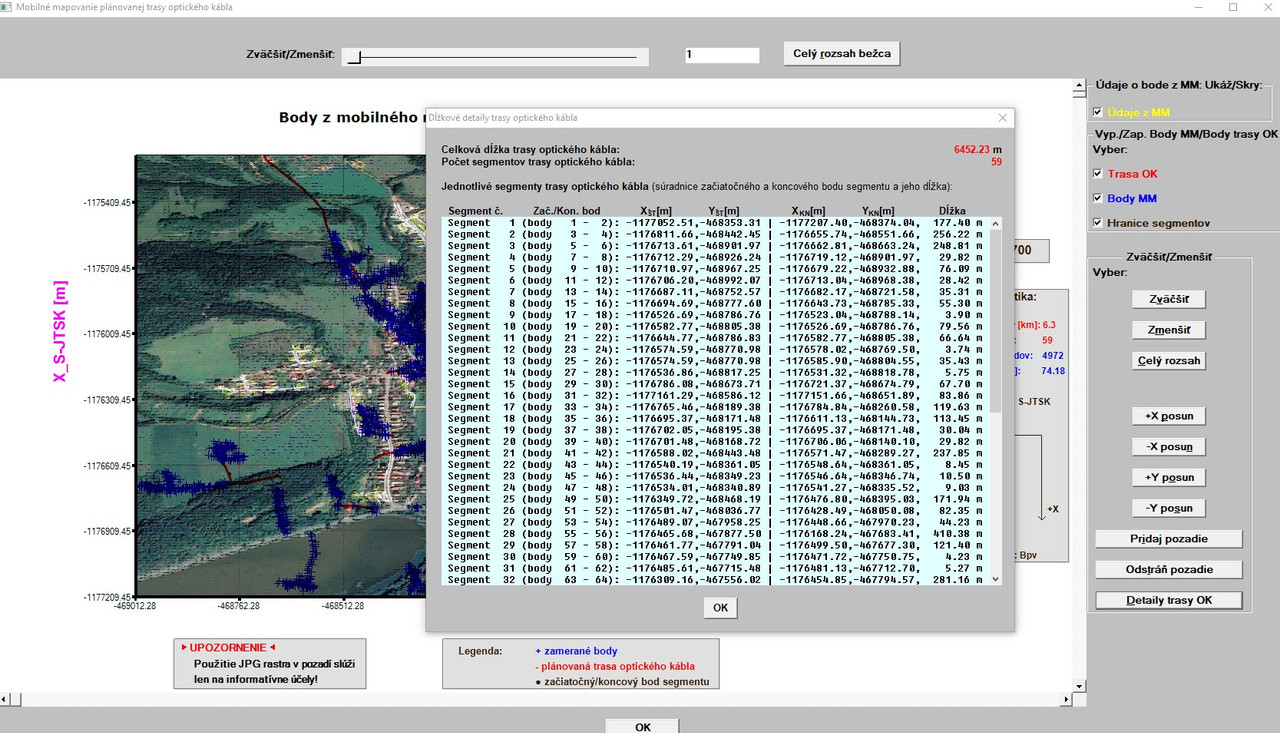I will double check the IF (TEST) conditions tomorrow.Martin_K

Joined: 09 Apr 2020
Posts: 227Posted: Thu Apr 08, 2021 12:32 pm    Post subject:Ken,

I found the problem! So, there is NO need to inspect my code.

1 - The test was put on wrong place in the code
2 - I used wrong index for X,Y coordinates in a DO loop!

The correct code is as follows (part of the %PL callback in the

 Code: ... IF (control(3) == 1) then               DO i = 1, ZAC_KON, 1   j = GET_PLOT_POINT@(Y_OK_ZK(i),X_OK_ZK(i),yy_pix,xx_pix)   INR_X = INRANGE_X (X_OK_ZK(i),X_MIN_NOW,X_MAX_NOW)   INR_Y = INRANGE_Y (Y_OK_ZK(i),Y_MIN_NOW,Y_MAX_NOW)     IF ((INR_X).AND.(INR_Y)) THEN       call draw_filled_ellipsed@(yy_pix, xx_pix, 2, 2, rgb@(51,25,0))       write (output_string(15),fmt(15)) i       call draw_characters@(output_string(15),nint(yy_pix+5),nint(xx_pix+5),rgb@(0,0,0))     END IF  END DO END IF ...

Now, everything works as expected!

Many thanks for your willingness to help me!Martin_K

Joined: 09 Apr 2020
Posts: 227Posted: Fri Apr 09, 2021 7:53 pm    Post subject:In the meantime, I added additional option to display the Point ID´s next to the graphical symbols of the points (blue points). This option is  by default  switched off, since it is useful to switch on it only when a part of the plot is sufficiently zoomed in (otherwise the plot is unreadable) and I accidentally found an issue which may or may not have a connection to the issue described by Ken in his post of Fri Apr 09, 2021 2:10 pm under the topic V8.71 %PL (I have 8.70).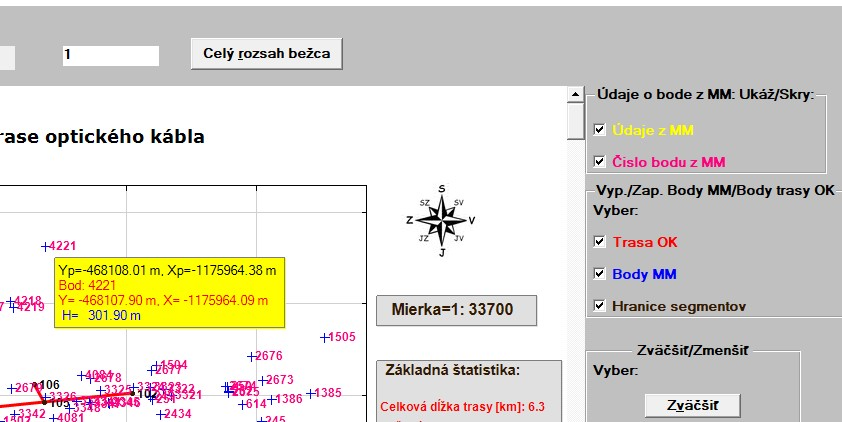In the plot area I have 2 options how to zoom in/out/to extents the plot: - Either using slider with its %DL callback - Or use a ZOOM IN (Zväčiť) button with its own callback I had a problem described in previous posts above which I nearly fully solved (originally, I thought that the problem is really fully solved). Today, I accidentally found that there still something remains unresolved: When I use the slider  everything works 100%. However, when I use ZOOM IN button, it works 100% problem free ONLY then, when it is used first without using previously the slider. As soon as I use the slider and then subsequently also the ZOOM IN button, then when using the ZOOM IN button all points run outside axes frame. When I then (after using ZOOM IN button) use the slider  everything is OK (slider functions problem free regardless whether the ZOOM IN button was used first or not and works also problem free when ZOOM IN button was used first). Till now, I was unable to catch the reason although I tried several different IF´s constructs for the ZOOM IN button in %pl callback in the section CB_REASON == PLOT_ADJUST. Simply, as soon as I use the slider, ZOOM IN button catches the problem with points running out of axes frame.Display posts from previous: All Posts1 Day7 Days2 Weeks1 Month3 Months6 Months1 Year Oldest FirstNewest First
 All times are GMT + 1 Hour Page 1 of 1